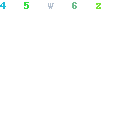### Mathematical Literacy: Bridging the Gap between Mathematical Literacy and Mathematical Understanding

Mathematical Literacy: Bridging the Gap between Mathematical Literacy and Mathematical UnderstandingFile Size:
4.74 MB
Teacher name(s):
Denise Buckley, Lyndsey Moriarty, Amanda Brosnan, Siobhan Ashe
School:
Mercy Secondary School, Mounthawk
Email: